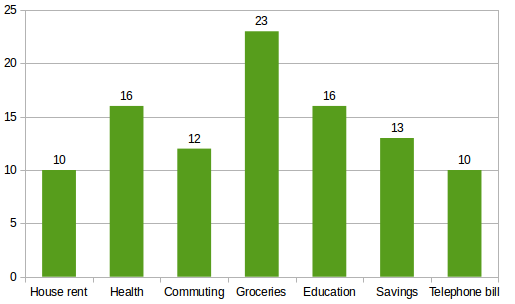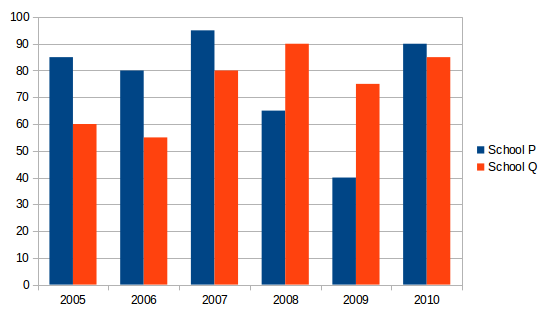## Data Interpretation For SBI PO : Set – 57

D.1-5) Study the bar chart carefully and answer the questions.

Percentage wise Breakup of spending Pattern of a family in a month.

Total Amount of salary = 600001) What is the amount spent by the family on Commuting?

a) Rs.9600

b) Rs.8400

c) Rs.7200

d) Rs.8000

e) None of these

c)

Amount spent by the family on commuting = 12% of 60000 = Rs.7200

2) What is the total amount spent by the family on telephone bill,health and education together?

a) 13800

b) 18600

c) 17400

d) 12800

e) None of these

e)

Amount spent on telephone bill = 10% of 60000

Amount spent on health = 16% of 60000

Amount spent on education = 16% of 60000

Total amount spent on these three fields =(10% + 16% +16%) of 60000

= 42% of 60000

= 25200

3) What is the respective ratio of amount spent by family on groceries to the amount spent on house rent?

a) 23:18

b) 13:28

c) 18:23

d) 23:13

e) None of these

e)

Amount spent on groceries = 23% of 60000

Amount spent on house rent = 10% of 60000

Required Ratio = 23 % of 60000/10% of 60000 =  23:10

4) Amount invested by the family in savings forms what percent of amount spent on health?

a) 123

b) 81.25

c) 120.50

d) 80.60

e) None of these

b)

Amount invested in Savings = 13% of 60000

Amount spent on health = 16% of 60000

Required Percentage = (13% of 60000/ 16% of 60000)*100= 13/16*100=81.25

5) Total Amount spent by the family on commuting and telephones bills together forms approximately what percent of the amount on groceries?

a) 153

b) 148

c) 135

d) 150

e) None of these

e)

Amount spent on commuting and telephone bills = (12+10)% of 60000

= 22% of 60000

Amount spent on groceries = 23% of 60000

required Percentage = ( 22% of 60000/23% of 60000 )*100=22/23*100=95.65

D.6-10) Study the following graph carefully to answer the questions that follow.

Number of students (in hundred) from Two different Schools who qualified in an Exam in Six different Years6) What was the approximate percent increase in the number of students who qualified in the exam from School Q in the year 2007 as compared to the previous year?

a) 30

b) 36

c) 45

d) 49

e) 26

c)

Number of students in school Q i 2007 = 8000

In the year 2006 = 5500

Increase = 8000-5500= 2500

Percentage increase = (2500/5500)*100= 45%

7) What was the respective ratio between the number of students who qualified in the exam from School P in the year 2005 and the number of students Who qualified in the exam school Q in the year 2008?

a) 13:18

b) 17:18

c) 17:19

d) 13:19

e) None of these

b)

Student qualified from school P in 2005 / Student qualified from school Q i 2008

85/90=17:18

8) What was the difference between the total number of Students Who qualified in the Exam in the year 2005 from both the schools together and the total number of Students  from school Q who qualified in the Exam overall the years together?

a) 30000

b) 30500

c) 29000

d) 29500

e) None of these

a)

Students qualified in 2005 from both the schools together =(85+60)*100=145*100=14500

Total number of students qualified from school overall the years together

= (60+55+80+90+75+85)*100=445*100=44500

Difference = 44500 – 14500 = 30000

9) Total number of students who qualified in the exam from School P overall the years together was approximately. what percentage of total number of students who qualified in the exam from both the schools together in the year 2006 and 2007 together?

a) 143

b) 159

c) 155

d) 165

e) 147

e)

Total number of students who qualified in the exam from School P over all the years together =(85+80+95+65+40+90)*100=455*100=45500

Total number of students who qualified in the exam from both the Schools together in the 2006 and 2007 years together=(80+55+95+80)*100 = 310*100=310000

Required Percentage = 45500/3100 *100= 147%

10) If 40% of the total Students Who qualified in the Exam from both the schools together overall the years are females, then what was the total number of males who qualified in the exams over all the years from both the Schools together?

a) 51000

b) 54000

c) 56000

d) 52000

e) None of these

b)

Total number of students who qualified in the exam overall the years from both the schools together =(85+60+80+55+95+80+65+90+40+75+90+85)*100=900+100=90000

Required number of males =90000 *60/100 = 54000